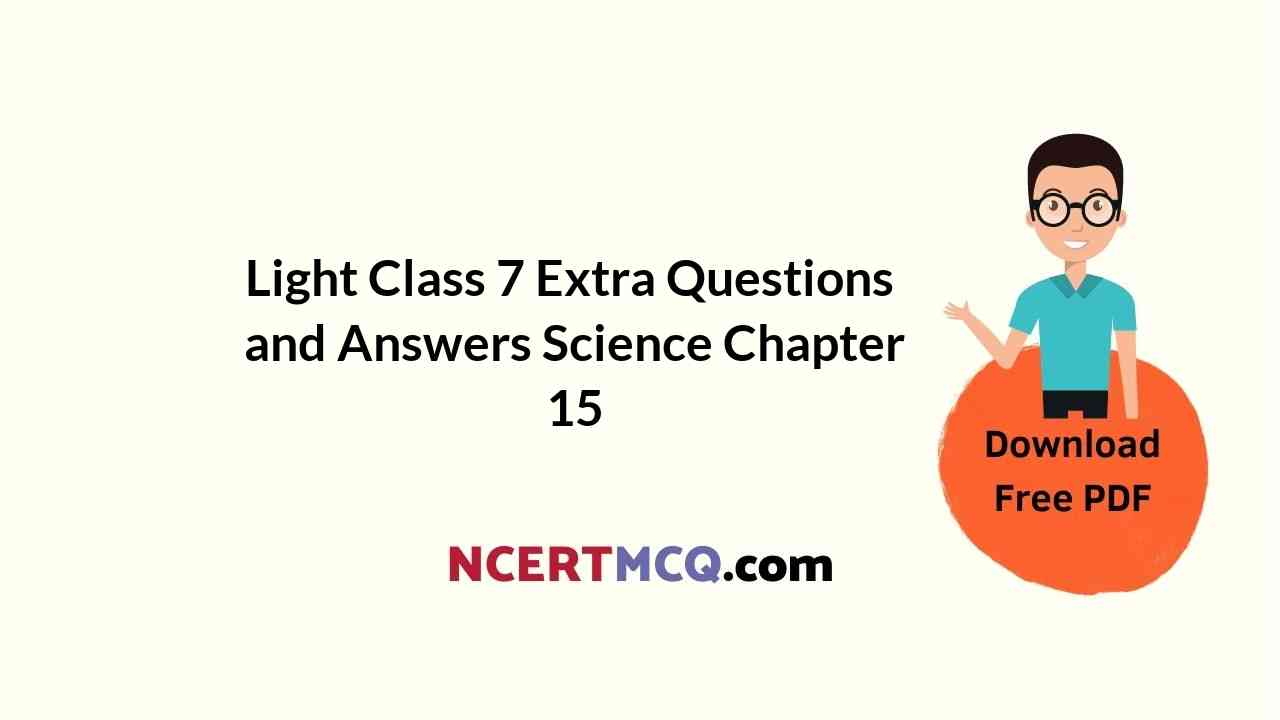Here we are providing Online Education for Light Class 7 Extra Questions and Answers Science Chapter 15 was designed by subject expert teachers. https://ncertmcq.com/extra-questions-for-class-7-science/

## Online Education for Class 7 Science Chapter 15 Extra Questions and Answers Light

Light Class 7 Extra Questions Question 1.
What is the path travelled by light?
Path travelled by a light is a straight line.

Light Class 7 Questions And Answers Question 2.
What is reflection?
The phenomenon of change of direction of light when it falls on a polished surface is called reflection.

Class 7 Light Extra Questions Question 3.
What makes objects visible to us?
Objects are visible to us only when light reflected from them reaches our eyes.

Class 7 Science Chapter 15 Light Extra Questions Question 4.
How is the word ‘AMBULANCE’ written on an ambulance?
The word ‘AMBULANCE’ is written laterally inverted on an ambulance. It appears as ‘aOKAJUHMA’.Class 7 Science Chapter 15 Extra Questions Question 5.
A hollow rubber ball is cut. Which are its convex and concave surfaces?
The inner surface of the cut ball is called concave and the outer surface is called convex.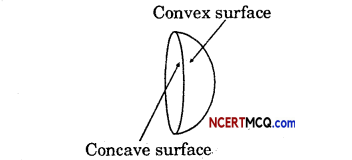Light Class 7 Extra Questions And Answers Question 6.
Draw concave and convex mirrors.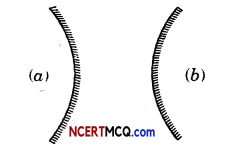Class 7 Science Light Extra Questions Question 7.
Draw convex and concave lens.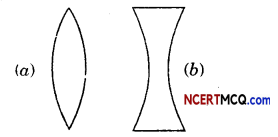Light Extra Questions Class 7 Question 8.
Draw a figure showing the splitting of white light by a prism.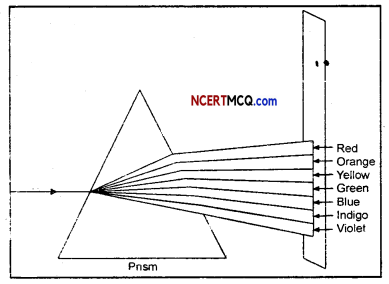Class 7 Science Chapter 15 Extra Question Answer Question 9.
Draw real image formation by a concave mirror.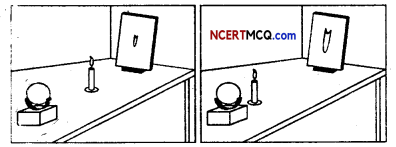Questions On Light For Class 7 Question 10.
Draw image formation by a convex mirror.Class 7 Science Chapter Light Extra Questions Question 11.
Draw real images formed by a convex lens.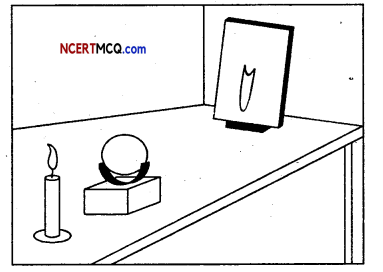Light Chapter Class 7 Extra Questions Question 12.
Draw virtual images formed by a convex lens.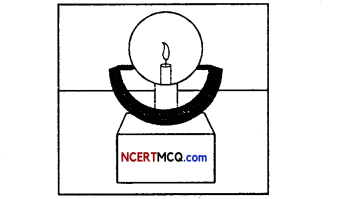Light Questions And Answers Class 7 Question 13.
How will you show that mixing of seven colours, gives white colour.
A circular cardboard disc of about 10cm diameter is taken. This disc is divided into seven segments. The seven rainbow colours are painted on these segments as shown in Fig. (a). A small hole is made at the centre of the disc.

The disc is fitted loosely on the tip of’ a refill of a ball pen so that the disc rotates freely [(Fig. (b)]. The disc is rotated in the daylight. When the disc is rotated fast, the colours get mixed together and the disc appears to be whitish.Extra Questions On Light Class 7 Question 14.
What is a virtual image? Give one situation where a virtual image is formed.
Image that cannot be formed on a screen is called virtual image. Plane mirror forms virtual image.Ch 15 Science Class 7 Extra Questions Question 15.
Can you now understand why the word ‘AMBULANCE’ is written inverted?
When the driver of a vehicle ahead of an ambulance looks in her/his rearview mirror, she/he can read ‘AAMBULANCE’ written on it and give way to it. It is the duty of every one of us to allow an ambulance to pass without blocking its way.

Class 7 Science Ch 15 Extra Questions Question 16.
Why was Boojho not able to see the can He flame through a bent pipe?
Light travels in a straight line. So, Light from the candle cannot travel through a bent pipe. That is why Boojho was not able to see the candle flame through a bent pipe.

Multiple Choice Questions

1. In a dark room, when we light a torch, the whole of room is illuminated. Which property of light made this possible?
(i) Light travels in a straight line.
(ii) Speed of light varies in different medium.
(iii) Light is reflected back from a polished surface.
(iv) Light is electromagnetic in nature.
(iii) Light is reflected back from a polished surface.

2. When a torchlight passing through a slit is incident on a mirror and when the torch is move slightly to either side, then
the reflected ray:
(i) moves away on other side.
(ii) moves back the same side.
(iii) no change occurs in the path of reflected ray
(iv) reflected ray superimposes the incident ray.
(i) moves away on other side.3. Which of the following is correct for plane mirrors?
(i) Image is always inverted.
(ii) Image is formed at the same distance behind the mirror as object is in front of the mirror.
(iii) Image is diminished.
(iv) Ithage is magnified.
(ii) Image is formed at the same distance behind the mirror as object is in front of the mirror.

4. When an object of height 5 cm is kept at a distance of 20 cm from a plane mirror. The height and distance of image from the mirror respectively are:
(i) 5 cm, 20cm
(ii) 10 cm. 20cm
(iii) 5 cm, 40cm
(iv) 10 cm, 40cm
(i) 5 cm, 20cm.

5. A candle is kept infront of a plane mirror, which of the following is correct?
(i) The image is erect and can be obtained get onto screen.
(ii) The image is erect and cannot be obtained onto screen.
(iii) The image is inverted and can be obtained onto screen.
(iv) The image is inverted & cannot be obtained onto screen.
(ii) The image is erect and cannot be obtained onto screen.6. Which of the following letters A, V, M, N, P, O, R change on looking in a plane mirror?
(i) A,VM
(ii) N,PR
(iii) V, M, O
(iv) A, V, P.
(ii) N,PR

7. Which of the following is correct for image formed by a plane mirror as compared to the object?
(i) The image is upside down.
(ii) The image is laterally inverted.
(iii) The image is magnified.
(iv) The imagé can be obtained on the screen.
(ii) The image is laterally inverted.

8. The image formed by the outer surface of the spoon is:
(i) inverted and larger than the object.
(ii) inverted and smaller than the object.
(iii) erect and larger than the object.
(iv) erect and smaller than the object.
(iv) erect and smaller than the object.

9. When the spoon is held at a distance, then the image formed by the Inner surface of the spoon is:
(i) inverted and larger than the object.
(ii) inverted and smaller than the object.
(iii) erect and larger than the object.
(iv) erect and smaller than the object.
(ii) inverted and smaller than the object.

10. When the spoon is hold close to object, then the image formed by the inner surface of the spoon is:
(i) inverted and larger than the object.
(ii) inverted and smaller than the object.
(iii) erect and larger than the object.
(iv) erect and smaller than the object.
(iii) erect and larger than the object.

11. A candle is kept at a distance from a concave mirror, the image formed is:
(i) virtual and erect
(ii) real and erect
(iii) virtual and invertecl
(iv) real and inverted.
(ii) real and erect.

12. When a candle is kept close to the concave mirror, the image formed on the screen is:
(i) real and inverted
(ii) virtual and inverted
(iii) real and erect
(iv) virtual and erect.
(iv) virtual and erect.13. Doctors use a mirror to see the enlarged view of ears, eyes, nose and throat. It is also used by Dentists to examine teeth. Name the mirror.
(i) Plane mirror
(ii) Concave mirror
(iii) Convex mirror
(iv) None of these.
(ii) Concave mirror.

14. The reflectors of torches, headlights of cars and scooters use a mirror to converge the light. Name the mirror.
(i) Plane mirror
(ii) Concave mirror
(iii) Convex mirror
(iv) None of these.
(ii) Concave mirror.

15. If the candle is placed near the convex mirror, the image formed will be:
(i) virtual and erect
(ii) virtual and inverted
(iii) real and erect
(iv) real and inverted.
(i) virtual and erect

16. An object is placed in front of a mirror X. The image of the object is always virtual, erect and dìminished in size Irrespective of the placing of the object. Can you Identify mirror X?
(i) Concave mirror
(ii) Convex mirror
(iii) Plane mirror
(iv) None of these
(ii) Convex mirror.

17. A mirror for rearview in the vehicle is used to see wide range view behind the vehicle. Which mirror should be preferred?
(i) Concave mirror
(ii) Convex mirror
(iii) Plane mirror
(iv) None off these.
(ii) Convex mirror.

18. Alens which converges the sunlight beam to a bright spot on a screen is a:
(i) convex lens
(ii) concave lens
(iii) plano-concave lens
(iv) pIano-convex lens.
(i) convex lens.

19. Given below are two lenses – concave and convex. What can we say about the image formed by them?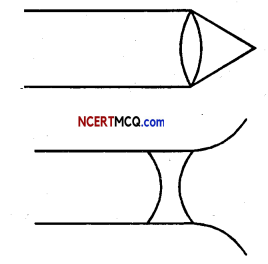(i) (a) virtual, (b) real
(ii) (a) virtual, (b) virtual
(iii) (a) real, (b) real
(iv) (a) real, (b) virtual.
(iv) (a) real, (b) virtual.20. The prism disperses light In spectrum of ……………………………. colours.
(i) six
(ii) seven
(iii) eight
(iv) ten.
(ii) seven.

21. As in case of Newton’s disc, when the seven colours of rainbow is pasted on a disc and if we make the disc to rotate rapidly. What colour do we get?
(i) Black
(ii) Nearly white
(iii) Greenish
(iv) Nearly brown
(ii) Nearly white.

Keywords:

→ Concave lens: A lens with thick edges and thin centre is called a concave lens.

→ Concave mirror: A mirror with inward curved reflecting surface is called a concave mirror.

→ Convex lens: A lens with thin edges and thick centre is called convex lens.

→ Convex mirror: A mirror with outward curved reflecting surface is called a convex mirror.

→ Erect image: Upright image.→ Magnified image: Image larger than the object.

→ Magnifying glass: A glass (lens) used to enlarge image.

→ Prism: A transparent material with three rectangular and two triangular surfaces used for obtaining spectrum of light.

→ Rainbow: A large arc of seven colours formed in the sky after the rain when the sun is low in the sky.

→ Real Image: Image that can be taken on a screen.

→ Rearview mirror: A mirror used in vehicles to see objects coming from behind.

→ Side mirror: A mirror used in vehicles to see objects coming from the right or left side.

→ Spherical mirror: Mirror with curved surface.

→ Virtual image: Image that cannot be taken on a screen.